Some teachers and parents use addition manipulatives to help students understand the basic addition facts. For example, adding groups of "Apple Jacks" (a breakfast cereal) by counting will quickly lead students to understand the concepts of addition. The sooner you can introduce base ten blocks to your students, the better. If you haven't already used them for counting, use them for basic addition and show students how regrouping works.

### Most Popular Addition Worksheets this Week

Disputably not a great way to learn addition facts, but undeniably a great way to summarize, addition facts tables are an invaluable resource in any home or school classroom.

### Five Minute Frenzy Charts

Five minute frenzy charts are 10 by 10 grids for addition fact practice. In each square, students write the sum of the column number and the row number.

Called mad minutes or timed drills by some, five minute frenzies are meant to be timed to add a little more excitement to practicing addition facts. They are ideally used to increase a student's ability to recall addition facts quickly which has all sorts of benefits later in their school life including preventing high school teachers from complaining about "how their students can't even add single-digit numbers without using a calculator."

A general goal to achieve would be to complete one chart in less than five minutes and score 98 percent or better, however, we recommend setting personal goals for students based on an initial test. If they are banging their head against the wall after a couple of minutes with only a few questions done, they really shouldn't be completing a timed addition facts drill at the moment. They still have some learning to do. We would recommend breaking out the manipulatives at this point. If they blast through the questions in 1.5 minutes and get almost all of them correct, they are probably ready for something a little more challenging.

Most people would agree that being able to add single-digit numbers quickly and in your head is an essential skill for success in math. The various addition worksheets in this section focus on skills that students will use their entire life. These worksheets will not magically make a student learn addition, but they are valuable for reinforcement and practice and can also be used as assessment tools.

A variety of strategies can be used to learn multi-digit addition; it isn't necessary to rely only on paper and pencil methods. Base ten blocks can help students conceptualize addition. Teaching students a mental left-to-right addition skill will help them in future math studies and life in general. E.g. 34 + 78 would be 30 + 70 = 100, 100 + 4 = 104, 104 + 8 = 112. Don't forget about using estimation with these worksheets.Add 1-digit and 2-digit numbers94 + 7 = ___Add 1-digit and 2-digit numbers (missing addend)94 + ___ = 103Complete one hundred (missing addend)___ + 85 = 100Adding a 2-digit and two 1-digit numbers4 + 2 + 17 =Adding a 3-digit and a 1-digit number865 + 5 = ___

### Whole tens

Add whole tens to 2-digit numbers20 + 39 = ___Add whole tens to 2-digit numbers (missing addend)20 + ___ = 59Adding two whole tens and two 1-digit numbers70 + 10 + 8 + 6 =__Adding whole tens (3 addends)60 + 50 + 40 = ___Adding whole tens (4 addends)60+50+40+30= ___

### Whole thousands

Adding whole thousands and whole hundreds7000 + 300 =Adding whole thousands to another number4,000 + 3,601 = ___

Adding two 2-digit numbers, no carrying

22
+ 23

38
+ 27

Adding two 3-digit numbers, no carrying

123
+ 234

216
+ 955

342
+ 123
+ 456

456
+ 123
+ 567
+ 890

5,432
+ 1,543

9,002
+ 1,004
+ 2,567

1,111
+ 2,222
+ 3,333
+ 4,444

##Addition WorksheetsHere is a graphic preview for all of the addition worksheets. These dynamically created addition worksheets allow you to select different variables to customize for your needs. The addition worksheets are randomly created and will never repeat so you have an endless supply of quality addition worksheets to use in the classroom or at home. Our addition worksheets are free to download, easy to use, and very flexible.

Click the image to be taken to that Addition Worksheet.##### 1 to 4 Digits With2 to 5 Addends Worksheets##### Adding Two Digit NumbersEnding in a Fixed Number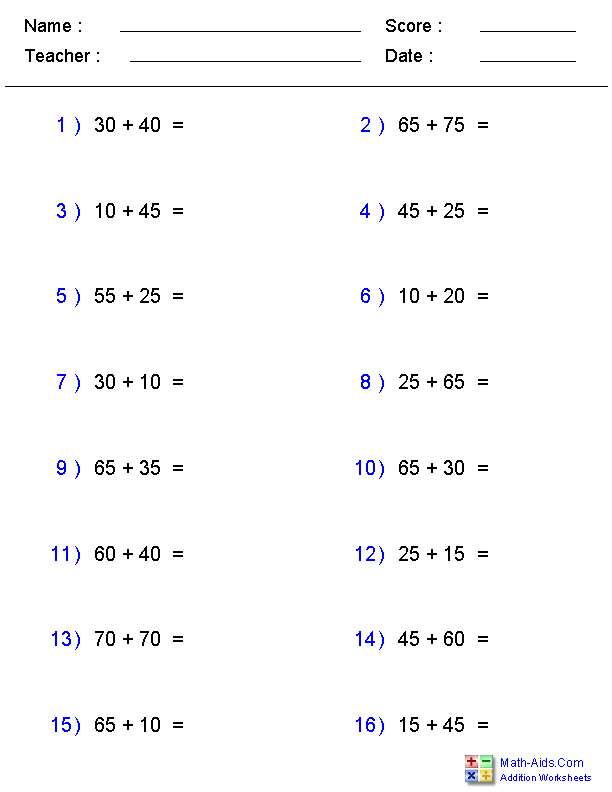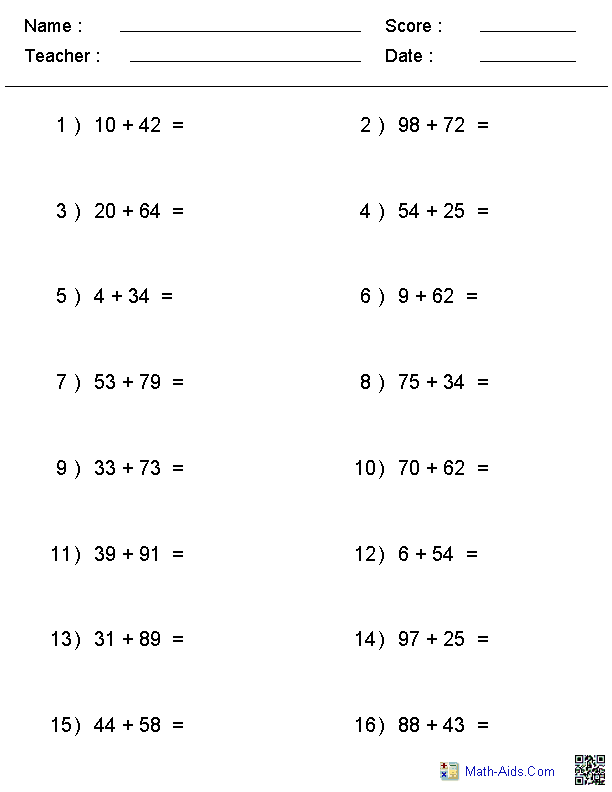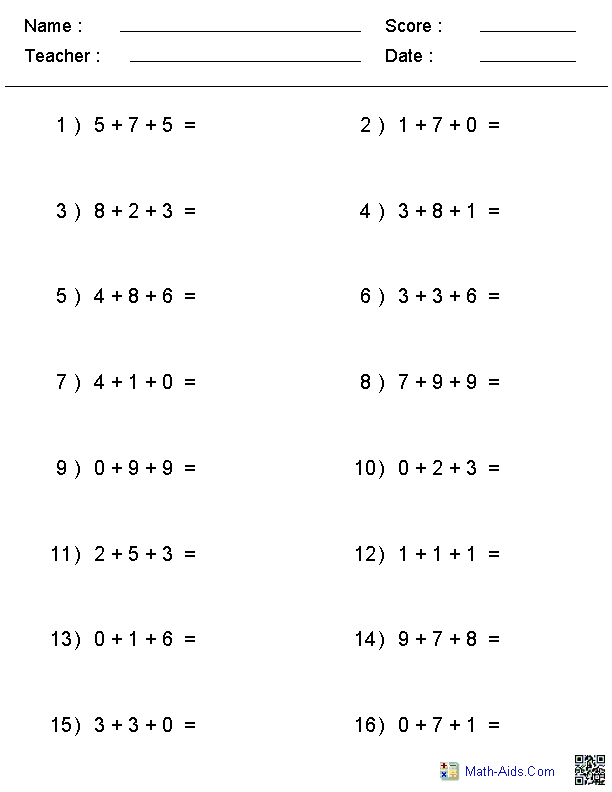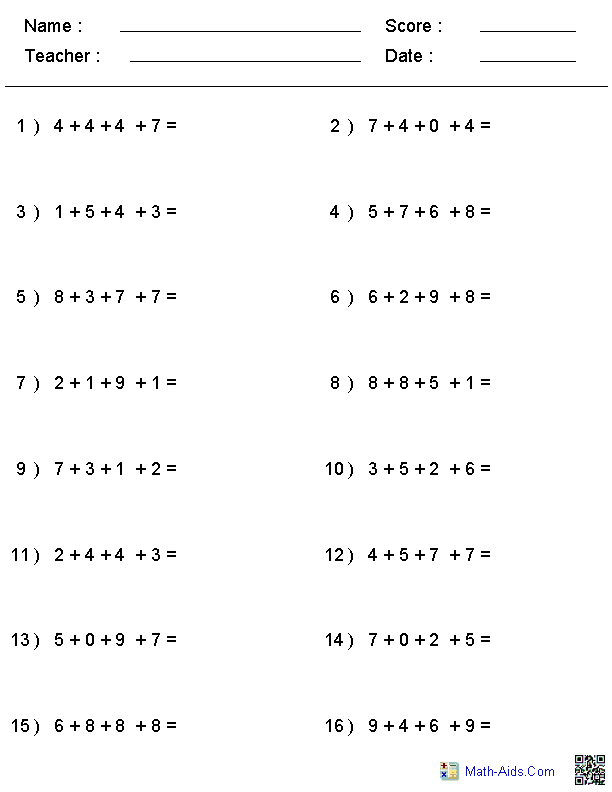##### 3 or 4 Digits - Horizontal Addition Worksheets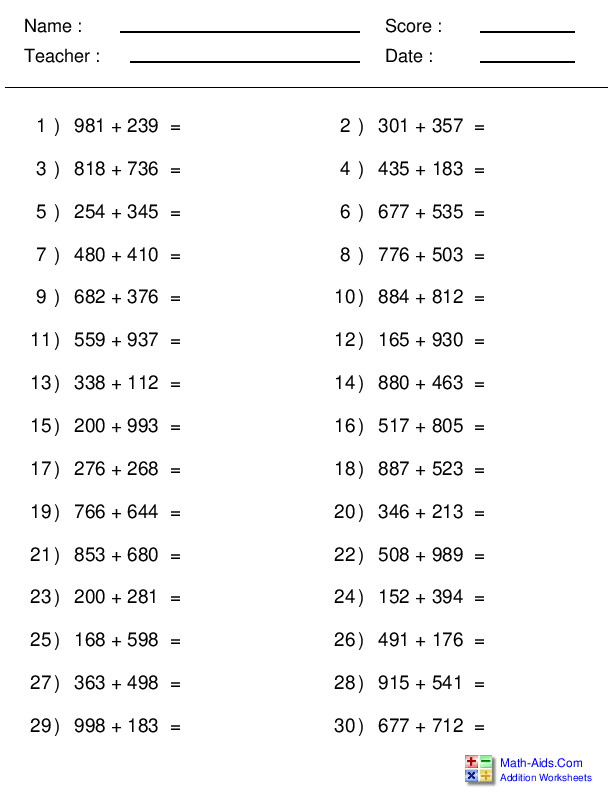##### 2, 3, or 4 Digits Worksheets2, 3, or 4 Addends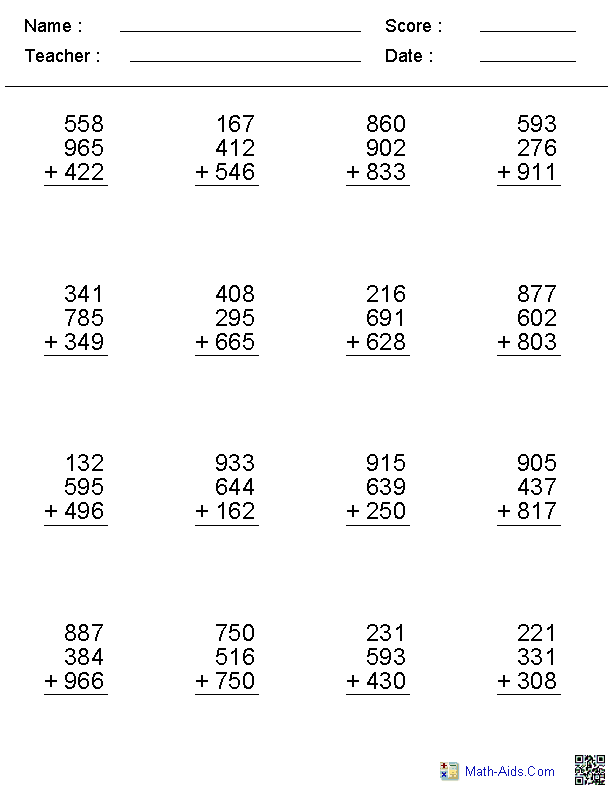##### 5, 6, or 7 Digits Worksheets2, 3, or 4 Addends##### Up to 4 Digits No RegroupingAddition Worksheets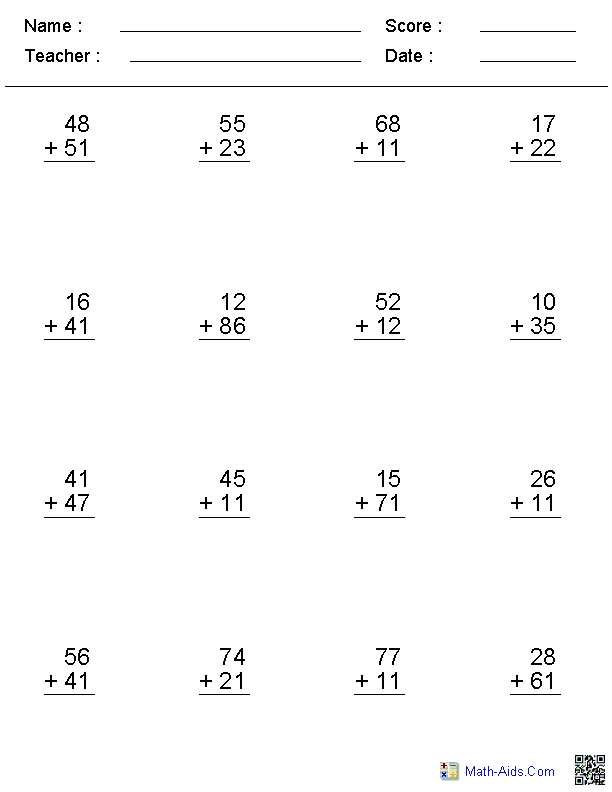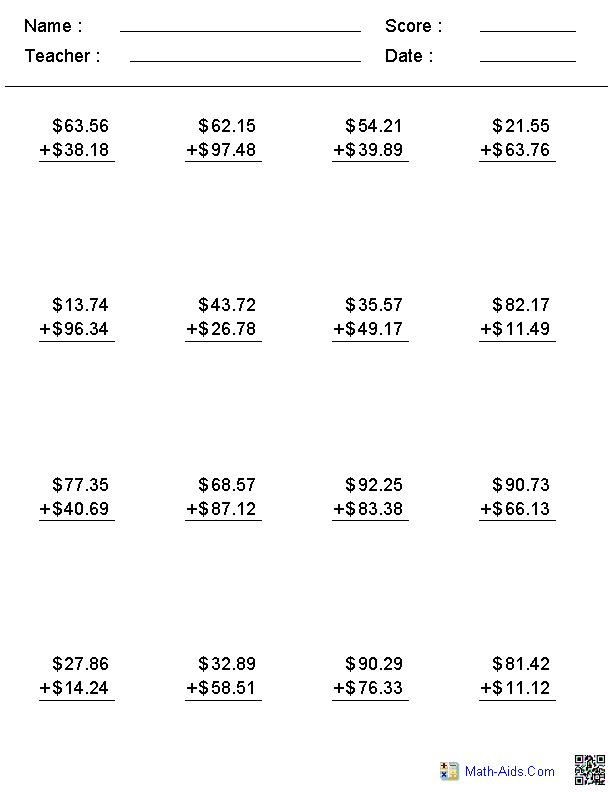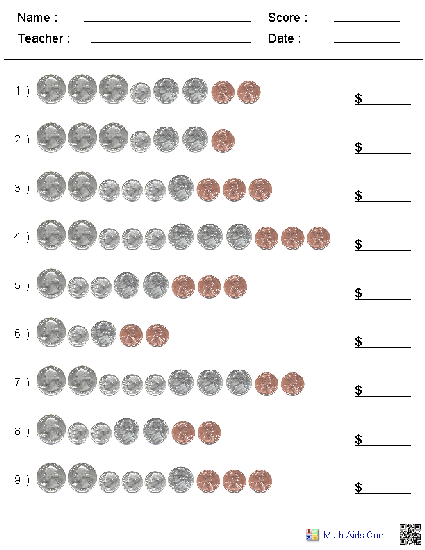##### 1, 3, or 5 Minute DrillsAddition Worksheets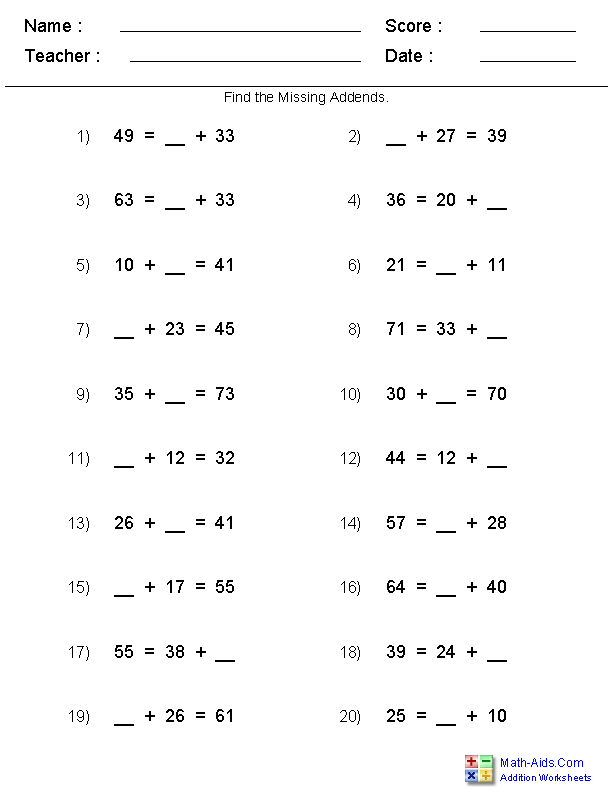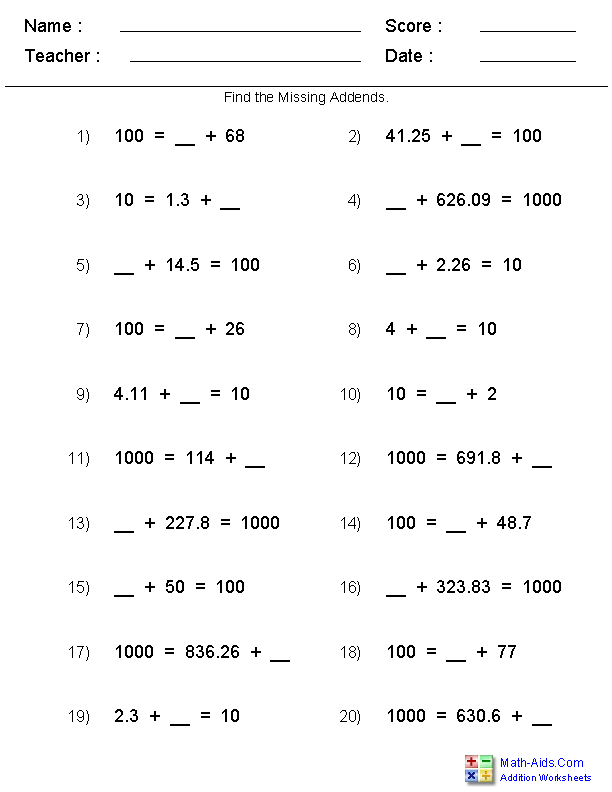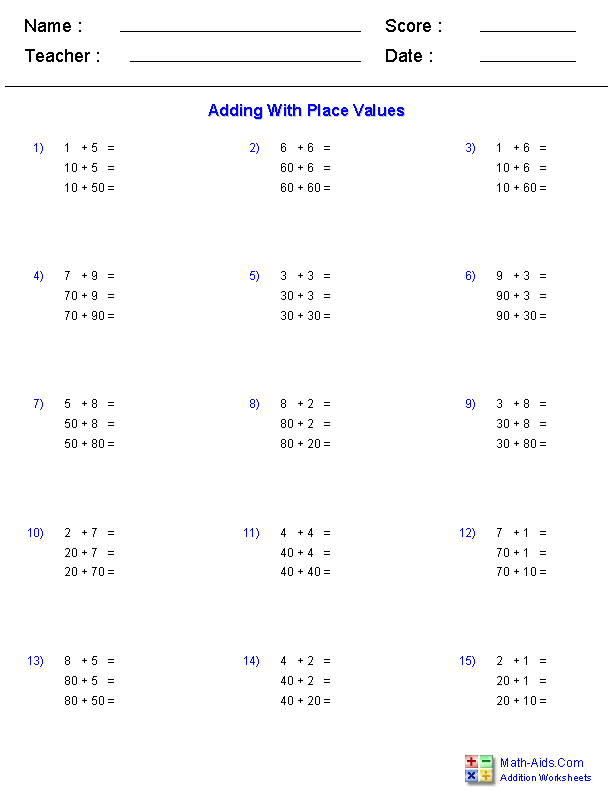##### 2, 3, or 4 Addendswith 1, 2, 3, or 4 DigitsAddition Worksheets##### 2, 3, 4, or 5 Addendswith 1, 2, 3, or 4 DigitsAddition Worksheets##### 2, 3, or 4 Addendswith 5, 6, or 7 DigitsAddition Worksheets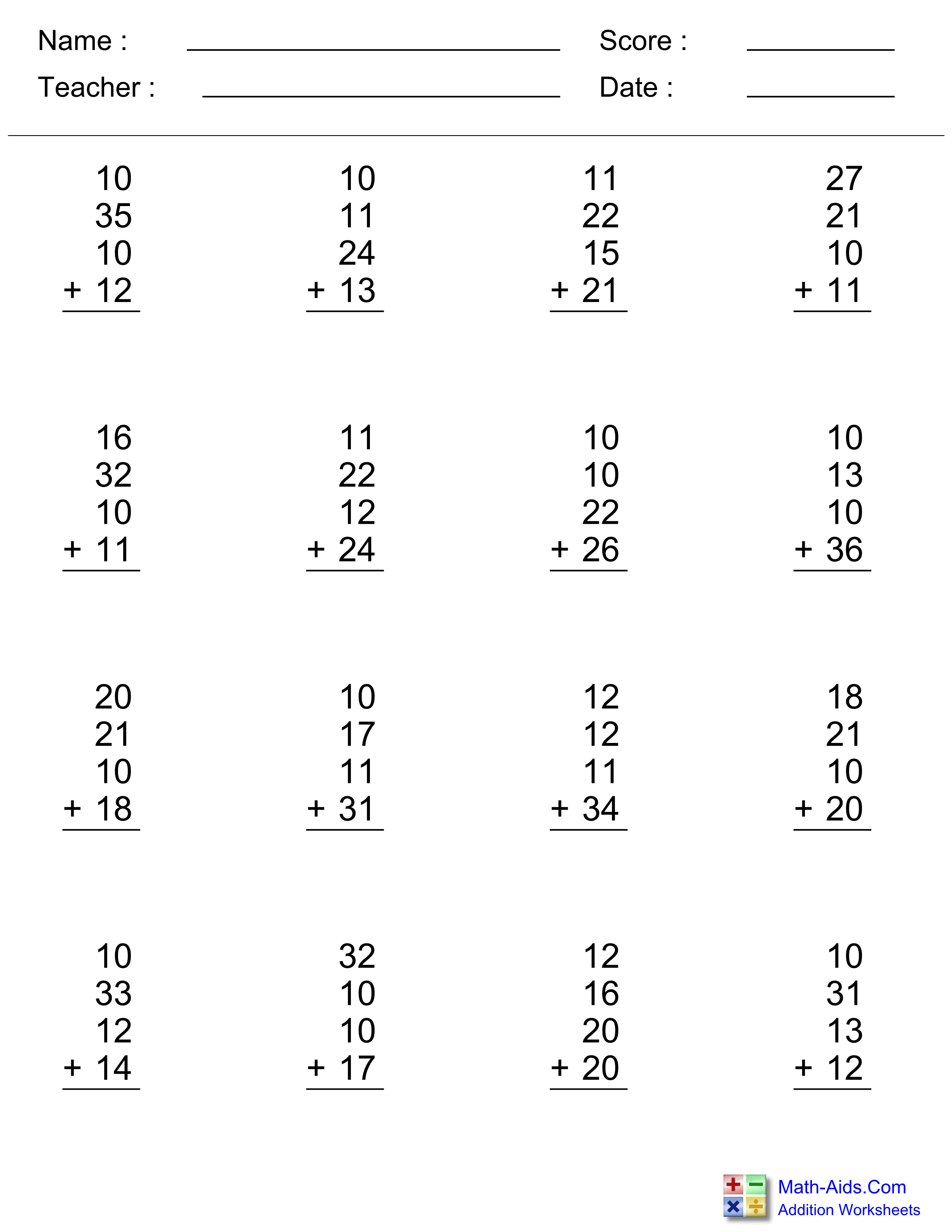### Detailed Description for All Addition Worksheets

These addition worksheets may be used to create five different addition table ranges starting at 0 and going through to 12. The addition tables worksheets may be created with all of the sums filled in or left blank. The numbers may be selected to be displayed in order or randomly shuffled for these worksheets.

These addition facts table charts are colorful and a great resource for teaching kids their addition facts tables. A complete set of printable addition facts tables for 1 to 12 will be created on these worksheets.

These single digit addition worksheets are configured for 2 addends in a vertical problem format. The numbers for each addend may be individually varied to generate different problem sets for these worksheets.

1 to 4 Digits Addition Worksheets
Vertical Format - 2 to 5 Addends

These addition worksheets may be configured for 1 to 4 digits as well as 2, 3, 4, or 5 Addends. You may select up to 30 addition problems per worksheet.

These addition worksheets will produce 2 addends problems in a vertical format where you may select numbers from zero to twenty to be used in the problems. The numbers for each addend may be individually varied to generate different sets of Addition problems. You may select up to 30 addition problems for these worksheets.

These addition worksheets will produce 2 addends problems in a vertical format where you may select numbers from zero to 99 to be used in the problems. The numbers for each addend may be individually varied to generate different sets of addition problems. You may select up to 30 addition problems for these worksheets.

These addition worksheets will produce vertical format addition problems within a selected sum. You may select the maximum sums and the number of problems to produce, or you may select the non repeating Kindergarten, 1st, or 2nd Grade set of unique problems. These special problem set will produce worksheets that will directly test the Common Core State Standards.

These addition worksheets will produce 12 vertical addition problems with dots to the right of each number to aid the children with the addition. You may select the numbers for the worksheets to be used from 0 to 9.

Adding Dot Figures to Ten Worksheets
Vertical Format or Horizontal - 2 Addends

These addition worksheets will produce 12 vertical or horizontal addition problems using dot figures to represent the numbers. You may select the numbers for the worksheets to be used from 0 to 10.

Adding Dot Figures to Twenty Worksheets

These addition worksheets will produce 10 horizontal addition problems using dot figures to represent the numbers up to twenty. The equations may be display under the dot figures if you select that option. You may select the numbers for the worksheets to be used from 0 to 20.

Adding Two Digit Numbers Ending in a Fixed Number Worksheets

These addition worksheets produces great worksheets that add two digit numbers together that end in a fixed number. The addends may be selected to be positive, negative or mixed numbers. You may select up to 30 addition problems per worksheet.

1 or 2 Digit Addition Worksheets

These addition worksheets may be configured for either 1 or 2 digit horizontal addition problems with 2 addends. The addends for the worksheets may be selected to be either positive, negative or mixed numbers.

1 or 2 Digit Addition Worksheets

These addition worksheets may be configured for either 1 or 2 digit horizontal addition problems with 3 addends. The addends for the worksheets may be selected to be either positive, negative or mixed numbers.

1 or 2 Digit Addition Worksheets

These addition worksheets may be configured for either 1 or 2 digit horizontal addition problems with 4 addends. The addends for the worksheets may be selected to be either positive, negative or mixed numbers.

3 or 4 Digits - Horizontal Addition Worksheets
This addition worksheet may be configured for either 3 or 4 digit horizontal addition problems with 2 addends. The addends may be selected to be positive, negative or mixed numbers.

These addition worksheets may be configured for adding doubles, double + 1, and double + 2 addition number sets in a vertical format. The addends for the worksheets may be selected from a number range of 0 to 20.

These addition worksheets may be configured for adding doubles, double + 1, and double + 2 addition number sets in a horizontal format. The addends for the worksheets may be selected from a number range of 0 to 20.

2, 3, or 4 Digits Addition Worksheets
Vertical Format - 2, 3, or 4 Addends

These addition worksheets may be configured for 2, 3, or 4 digits as well as 2, 3, or 4 addends addition problems. You may select between 12 and 30 problems for these worksheets.

5, 6, or 7 Digits Addition Worksheets
Vertical Format - 2, 3, or 4 Addends

These addition worksheets may be configured for 5, 6, and 7 digits as well as 2, 3, and 4 addends addition problems. You may select between 12 and 20 problems for these worksheets.

Up to 4 Digits No Regrouping Addition Worksheets
Vertical Format

These addition worksheets are great for problems that do not require regrouping. The problems may be configured with up to 4 digits and 2 addends. The no regrouping option may be switched off if some regrouping is desired. The problem format is vertical and you may select up to 30 addition problems per worksheet. The no regrouping option may be switched off for these worksheets if some regrouping is desired.

Vertical Format - 2, 3, or 4 Addends

These money addition worksheets may be configured for up to 4 digits as well as 2, 3, and 4 addends. The currency symbol may be selected from Dollar, Pound, Euro, and Yen for these worksheets.

Vertical Format - 2 or 3 Addends

These money addition worksheets may be configured for no regrouping, for up to 4 digits as well as 2 or 3 addends. The currency symbol may be selected from Dollar, Pound, Euro, and Yen for these worksheets.

These addition worksheets are great for teaching children to add up coins. Quarters, Dimes, Nickels, & Pennies may be selected for each problem. You may select between 3 and 7 for the maximum number of coins used on the worksheets.

Vertical Format

1, 3, or 5 minute drill addition worksheets contain all of the single digit addition problems on one page. A student who has memorized all of the single digit addition problems should be able to work out these worksheets in the allowed time.

Vertical Format

This worksheet will generate advanced addition drills as selected by the user. The user may select from 256 different addition problems from addition tables ranging from 0 to 15. The user may also select a 1 minute drill of 20, a 3 minute drill of 60 problems, a 5 minute drill of 100 problems, or a custom drill with ranges from 20 to 100 problems and times of 1 to 5 minutes.

These addition worksheets are a great introduction for algebra concepts. You may select various types of characters to replace the missing addends. The format on these worksheets are horizontal and the numbers used may be selected from the range of 0 to 99.

These addition worksheets are a great introduction for building missing addend problems. You may select various forms for the addition problems and the range of numbers to use. The formats of the problems are horizontal and you may select 12, 16, 20, 24 or 30 addition problems per worksheet.

These addition worksheets are a great for building missing addend problems that add up to multiples of ten. You may select various forms for the addition problems and the range of numbers to use. The formats of the problems are horizontal and you may select 12, 16, 20, or 24 addition problems per worksheet.

These multiple digit addition worksheets are configured for 2 addends in a vertical problem format. The missing digits on these addition worksheets are randomly selected to challenge the children in solving the addition problems. The number of digits on these worksheets may be varied between 2 and 4.

These addition worksheets are great for teaching children to add irregular units of measurement. The addition problem may be selected to include Feet & Inches, Pounds & Ounces, Hours & Minutes, and Minutes & Seconds for these addition worksheets. These worksheets will produce 15 problems per worksheet.

These addition worksheets are great for practicing adding together two measurements of feet with fractional inches. These worksheets will use 1/2's, 1/4's, 1/8's. 1/16's and there is an option to select 1/32's and 1/64's.

Vertical Format - 2, 3, or 4 Addends

These addition worksheets may be configured for 1, 2, or 3 digits on the right of the decimal and up to 4 digits on the left of the decimal as well as 2, 3, and 4 addends addition problems for these worksheets.

These addition worksheets will produce problems to practice addition with numbers of differing place values. Each problem will have 3 separate equations: Adding 2 single digit numbers, adding a single digit and double digit number, and adding 2 double digit numbers. You may select the addends and number of problems per worksheet.

Vertical Format - 1, 2, 3, or 4 Digits

These addition worksheets may be configured for different combinations of 2, 3, or 4 Addends with 1, 2, 3, or 4 digits. You may select up to 30 addition problems per worksheet.

2, 3, 4, or 5 Addends
Vertical Format - 1, 2, 3, or 4 Digits

These addition worksheets may be configured for different combinations of 2, 3, 4, or 5 Addends with 1, 2, 3, or 4 digits. You may select up to 30 addition problems per worksheet.

Vertical Format - 5, 6, or 7 Digits

These addition worksheets may be configured for different combinations of 2, 3, or 4 Addends with 5, 6, or 7 digits. You may select up to 30 addition problems per worksheet.

These addition worksheets may be configured for visual addition problems with geometric shapes. These addition worksheets may be configured for their color scheme as well as their problem layout and difficulty.

These addition worksheets may be configured for adding doubles, double + 1, and double + 2 addition number sets in a vertical format. The problems are represented with dots to the right of each number. The addends for the worksheets may be selected from a number range of 0 to 20.

Vertical Format

These addition worksheets may be configured for 2, 3, or 4 addends and allows for the selection of which columns require regrouping. You may select up to 20 addition problems per worksheet.One of them took out a barrel. Serega and I went into the corner, Lenka looked at them, then at us with huge eyes. - Guys, we have a wet, neo-fucked whore here.

Miracle !, Sergei said, kissing the girl: he had no prejudices about kissing after a blowjob: Next time, it's my turn to caress you there with my tongue. " Ira smiled even wider. After graduating from work at the brewery, and having received the calculation, my friend Viktor and I gathered to.

Go to rest on the seas. However, it happened as in a proverb.

I especially like what I now feel through your trousers. She smiled a little. - And it's all yours.

### Now discussing:

Here you rodents. Even wine is your enemy. Yes, I ran into it. A good scuffle was required. But even Lasha missed my hint, looking at Julia, in whose ass two dicks were squirming.

1125 1126 1127 1128 1129